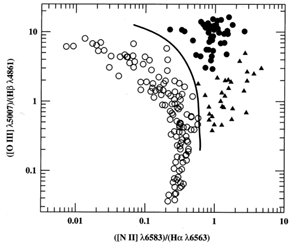### BPT diagram

``Baldwin, Phillips & Terlevich'' (BPT) diagrams demonstrate how LINERs can be distinguished from normal H II regions and normal AGNs (Seyferts and QSOs) on the basis of their [O III]5007 / H, [N II]6583 / H, and [S II]6716, 6731 / Hflux ratios. Here it is seen that the Seyfert 2s have high values of each ratio. H II regions define a locus of lower values which does not overlap with the region of parameter space occupied by the Seyferts. The LINERs can be distinguished from the Seyfert 2s by their low values of [O III]5007 / Hrelative to [N II]6583 / H, and from the H II regions by their larger values of [N II]6583 / H.Figure 2.3. A diagnostic (or BPT) diagram for emission-line galaxies. The vertical axis is the [O III]5007 / Hflux ratio and the horizontal axis is the [N II]6583 / Hflux ratio. Both ratios are based on lines close in wavelength and are therefore nearly reddening independent. The open circles are for H II regions and similar sources that are clearly ionized by hot stars. The closed circles are narrow-line AGNs (Seyfert 2s and NLRGs) which are ionized by ``harder'' continua (i.e., with a greater fraction of high-energy photons, such as a power-law spectrum); the solid line is an empirical division between these two classes of object. The triangles represent LINERs, which can be distinguished from H II regions by higher values of [N II]6583 / H, and from Seyfert galaxies by lower values of [O III]5007 / H, as can also be seen in Figure 2.1. Based on a similar diagram from Osterbrock (1989), p. 346. Figure courtesy of R. W. Pogge, based on data from Veilleux and Osterbrock (1987).

Adapted from B.M. Peterson An Introduction to Active Galactic Nuclei, Cambridge University Press, (1997)